Home > AC > Chapter 11 > Lesson 11.1.5 > Problem11-61

11-61.
1. Find the inputs for the following relations with the given outputs. If there is no possible input for the given output, explain why not. Homework Help ✎

1.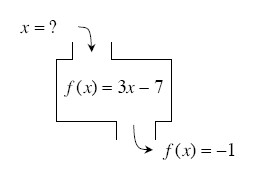2.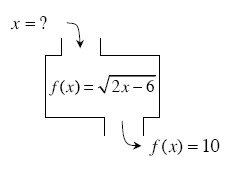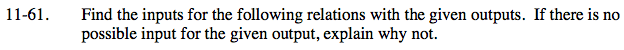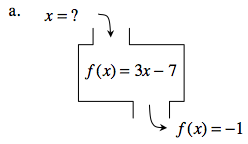Write out the equation.

−1 = 3x − 7

Solve for x.

6 = 3x

2 = x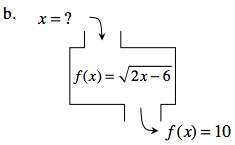Follow the steps in part (a).